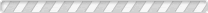# Concentrated Volumetric Solutions (Cvs)

 Code Product Name CAS No. MSDS COAs
 AMP003 Ammonium Ferrous Sulphate Solution 0.1 mol/lAMP005 Ammonium Thiosulphate N/10 Solution (0.1 mol/l Citrisol)AMP006 Barium Chloride Solution 0.05mol/LAMP020 E.D.T.A Disodium Salt Solution 0.01 mol/lAMP011 E.D.T.A. Disodium Salt 0.1 mol/L (0.1 N)AMP023 Hydrochloric Acid 0.02 mol/L (0.02N)AMP021 Hydrochloric Acid 0.1 mol/LAMP025 Hydrochloric Acid 0.5 mol/L (0.5N)AMP031 Hydrochloric Acid 1 mol/LAMP037 Hydrochloric Acid Solution 0.01mol/L (0.01N)AMP041 Iodine Solution 0.05 mol/LAMP056 Nitric Acid Solution 0.1mol/L (0.1N)AMP060 Oxalic Acid Solution 0.005mol/L (0.01N)AMP061 Oxalic Acid Solution 0.05 mol/LAMP065 Potassium Bromate 1/60 mol/l (0.1N)for 1000 ml SolutionAMP068 Potassium Bromide 0.1 mol/l (0.1N)for 500 ml SolutionAMP071 Potassium Dichromate Solution 0.1 NAMP076 Potassium Hydroxide 0.1mol/L (0.1N)AMP074 Potassium Hydroxide Solution 1mol/L (1N)AMP081 Potassium Permanganate Solution 0.02 mol/LAMP091 Silver Nitrate Solution 0.1 mol/LAMP101 Sodium Carbonate N/10AMP106 Sodium Chloride 0.1M (0.1N) SolutionAMP111 Sodium Hydroxide Solution N/1AMP115 Sodium Hydroxide Solution N/10AMP121 Sodium Thiosulphate Solution 0.1 mol/L (0.1 N)AMP123 Sulphuric Acid Solution 0.005mol/L (0.01N)AMP131 Sulphuric Acid Solution 0.1NAMP126 Sulphuric Acid Solution 0.5 mol/LAMP201 Zinc Sulphate 0.1M for 500 ml Solution